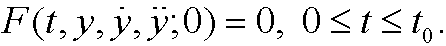2. The main idea behind perturbation methods.

Consider the second order differential equationwhereis a small parameter,We will try to solve it by inserting the perturbation seriesand then find y0(t), y1(t), y2(t),…
We can for instance useas a good approximation to the solution of the differential equation. Here y0(t) is the leading term, the solution of the unperturbed problemThe termsare higher order terms which usually are “small”.

Remark: The unperturbed problem can in many cases be solved exactly.